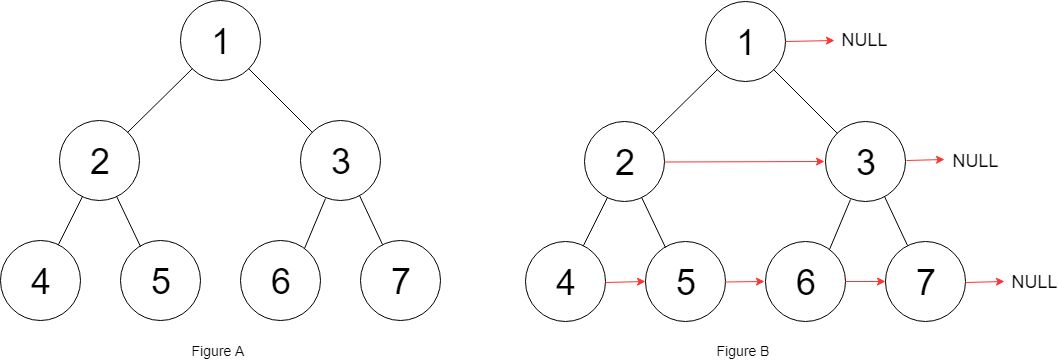116. Populating Next Right Pointers in Each Node

#### QUESTION:

You are given a perfect binary tree where all leaves are on the same level, and every parent has two children. The binary tree has the following definition:

struct Node { int val; Node *left; Node *right; Node *next; } Populate each next pointer to point to its next right node. If there is no next right node, the next pointer should be set to NULL.

Initially, all next pointers are set to NULL.

Example 1:``````Input: root = [1,2,3,4,5,6,7]
Output: [1,#,2,3,#,4,5,6,7,#]
Explanation: Given the above perfect binary tree (Figure A), your function should populate each next pointer to point to its next right node, just like in Figure B. The serialized output is in level order as connected by the next pointers, with '#' signifying the end of each level.
``````

Example 2:

``````Input: root = []
Output: []
``````

Constraints:

``````The number of nodes in the tree is in the range [0, 212 - 1].
-1000 <= Node.val <= 1000
``````

Follow-up:

``````You may only use constant extra space.
The recursive approach is fine. You may assume implicit stack space does not count as extra space for this problem.
``````

#### SOLUTION:

``````/**
* Definition for a Node.
* public class Node {
*     public var val: Int
*     public var left: Node?
*     public var right: Node?
*	   public var next: Node?
*     public init(_ val: Int) {
*         self.val = val
*         self.left = nil
*         self.right = nil
*         self.next = nil
*     }
* }
*/

class Solution {
func connect(_ root: Node?) -> Node? {
var queue: [Node] = []
var arr:[Node] = []
if root == nil {
return root
}
queue.append(root!)
while !queue.isEmpty {
let tmp = queue.removeFirst()
arr.append(tmp)
if tmp.left != nil {
queue.append(tmp.left!)
}
if tmp.right != nil {
queue.append(tmp.right!)
}
}
var next = 2
var index = 0
for (i, node) in arr.enumerated() {
if i == index {
node.next = nil
index += next
next *= 2
} else {
node.next = arr[i+1]
}
}
return root
}
}
``````